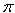Name: Nicole Who is asking: Other Level of the question: All Question: How many gallons of water are in a 24' X 4' round swimming pool? Hi Nicole. To solve this problem you need to calculate the volume of the swimming pool in cubic feet, then convert that to gallons. To calculate the volume of the swimming pool, you would first use the formula A =r2 to find the surface area of the top of the pool, then multiply that by the depth. Since your swimming pool is 24 feet across, the radius is half of that. So the volume in cubic feet is 4ft x122. To convert to imperial gallons, you'd multiply this by 6.23, because that's the number of gallons in one cubic foot. American gallons are smaller than imperial gallons, so if that's the unit you want to use, the conversion factor is 7.48 instead of 6.23. Hope this helps, Stephen La Rocque.